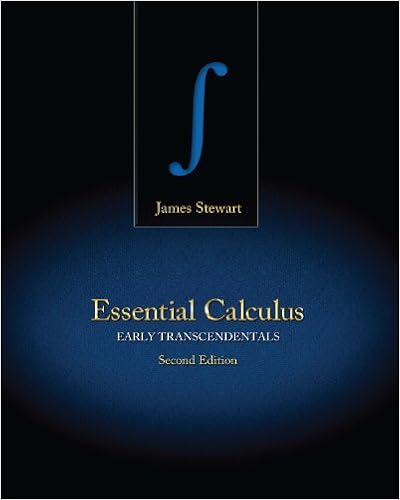Assign3 - 137 - F18 SOLUTIONS.pdf - MATH 137 PRACTICE...

• Homework Help
• 7

This preview shows page 1 - 3 out of 7 pages.

We have textbook solutions for you!
The document you are viewing contains questions related to this textbook.The document you are viewing contains questions related to this textbook.
Chapter 1 / Exercise 29
Essential Calculus: Early Transcendentals
StewartExpert Verified
MATH 137 PRACTICE ASSIGNMENT 3 SOLUTIONS 1. Assuming lim n →∞ a n = lim n →∞ b n = 0 where a n 0 and b n 0, determine lim n →∞ ( a n sin( n ) + b n cos( n )) .
We have textbook solutions for you!
The document you are viewing contains questions related to this textbook.The document you are viewing contains questions related to this textbook.
Chapter 1 / Exercise 29
Essential Calculus: Early Transcendentals
StewartExpert Verified
2. Let’s examine how absolute values and limits interact. (a) The statement If lim n →∞ | a n | = | L | then lim n →∞ a n = L is false in general. Provide a counter-example. (b) The statement If lim n →∞ a n = L then lim n →∞ | a n | = | L | is true. Show this using the definition of limits. Hint: || a | - | b || ≤ | a - b | (Not required: Can you show that the Hint is true?) (c) Is the statement If lim n →∞ | a n | = 0 then lim n →∞ a n = 0 true? If so, argue why, if not, provide a counterexample. Solution
•••